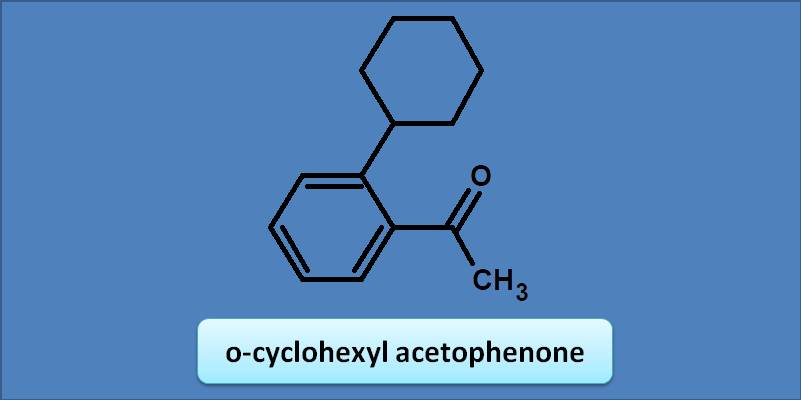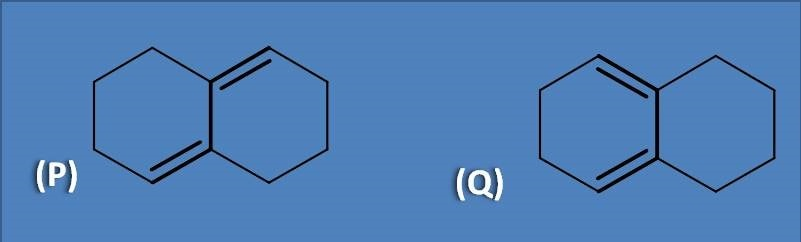# MCQ on UV-Visible spectroscopy: Page-3

1. Calculate the λmax for the following compound(A) 243 nm

(B) 250 nm

(C) 230 nm

(D) 217 nm

Here the base value of the benzoic acid is 230 nm. Amine group at meta position acts as auxochrome with an increment of 13 nm. Therefore the total calculated value for lambda max will be 230 + 13=243 nm. img

2. The l max for o-cyclohexyl acetophenone is(A) 230 nm

(B) 245 nm

(C) 240 nm

(D) 249 nm

Acetophenone has bas value as 246 nm. Any alkyl or cycloalkyl group at ortho position adds an increment of 3 nm. Hence the total calculated value will be 246 +=249 nm

Given below are the dienes with different configuration.(A) P is cisoid and Q is transoid

(B) P is transoid and Q is cisoid

(C) Both P and Q are cisoid

(D) Both P and Q are transoid

Cisoid are the cyclic compounds that has double bonds arranged in a fashion that both double bonds are same side to the sigma bond connecting them. On the other hand, transoids will have double bounds on opposite side to the sigma bond. Therefore homoannular dienes are cisoids while heteroannular dienes are transoids.

4. In the above question, the base value of Q is

(A) 217 nm

(B) 214 nm

(C) 208 nm

(D) 253 nm

The structure at option Q is cisoid which shows more absorption with base value at 253 nm while transoid absorbs at 214 nm.

5. Choose the right statement

Homoannular diene is called as transoid

Cisoid shows less intense absorption

The base value of transoid is 214 nm

Exocyclic double bond causes a bathochromic shift of 30 nm

Cisoid has both of the double bonds within the same ring hence called as homoannular diene. Similarly, transoid has double bonds each in one ring hence called as heteroannular diene.